# Highlight Cells That End with Specfic Characters

This post will guide you how to highlight values that end with a specific character or text string in excel. How do I highlight cells that end with a string using the conditional formatting feature in excel.

Assuming that you want to highlight cells that end with the text string “excel” in the range A1:B4, you can refer to the following tow method.

## Highlight Cells that End with a String using Conditional Formatting

If you want to highlight cells that end with a specific text string, you can create a simple formula based on the COUNTIF function to apply conditional formatting. And this formula will check if the selected cells match the given criteria that end with “excel”. Just do the following steps:

#1 select the cells of range that you want to highlight.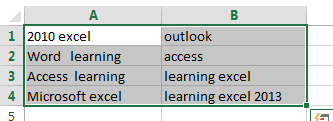#2 go to HOME tab, click Conditional Formatting command under Styles group, then select New Rule… from the drop down list. The New Formatting Rule dialog will appear.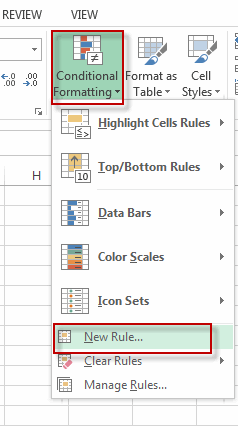#3 select Use a formula to determine which cells to format in the Select a Rule Type section. Then type the formula =COUNTIF(A1,”*excel”) into the text box of  Format values where this formula is true: . Click Format… command, the Format Cells dialog will appear.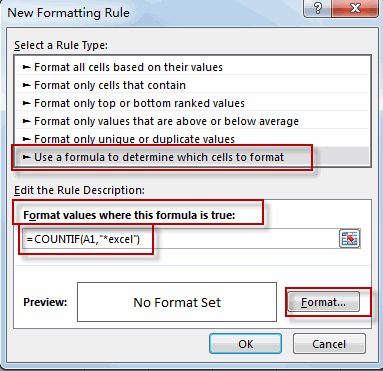#4 click Fill tab, choose one background color as you need. Click OK.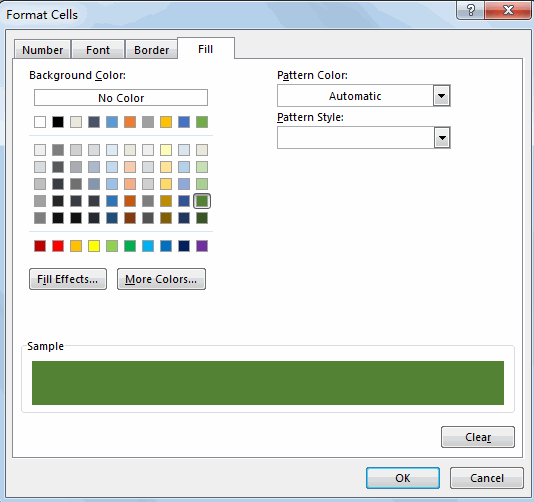#5 click OK. You will see that all cells that end with “excel”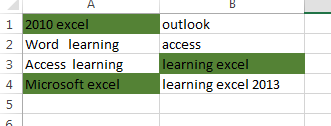Note: the COUNTIF function is not case-sensitive, so if you want to check the cells in case sensitive, you can use a more complicated formula based on the RIGHT function, the EXACT function and the LEN function. Just type the following formula in the above step 3.

=EXACT(RIGHT(A1,LEN(“excel”)),”excel”)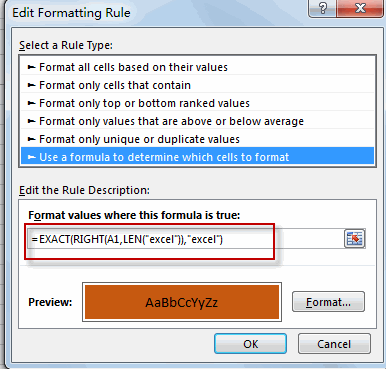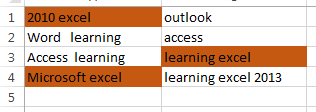The RIGHT function will extract the text from the most right of each cell in the selected cell, and the LEN function will return the length of the specific text string and then passed it into the RIGHT function. The EXACT function will compare the extracted text by RIGHT function to the given text string, if True, then return the TRUE value. Otherwise, it returns FALSE.

### Related Functions

• Excel COUNTIF function
The Excel COUNTIF function will count the number of cells in a range that meet a given criteria. This function can be used to count the different kinds of cells with number, date, text values, blank, non-blanks, or containing specific characters.etc.= COUNTIF (range, criteria)…
• Excel EXACT function
The Excel EXACT function compares if two text strings are the same and returns TRUE if they are the same, Or, it will return FALSE.The syntax of the EXACT function is as below:= EXACT (text1,text2)…
• Excel RIGHT function
The Excel RIGHT function returns a substring (a specified number of the characters) from a text string, starting from the rightmost character.The syntax of the RIGHT function is as below:= RIGHT (text,[num_chars])…
• Excel LEN function
The Excel LEN function returns the length of a text string (the number of characters in a text string).The syntax of the LEN function is as below:= LEN(text)…